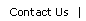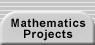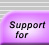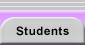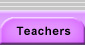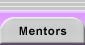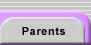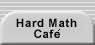# INTRODUCTORY EXPLORATIONS

The Teacher Handbook includes activities for teaching the skills of doing mathematics research. Although students can, and should, study these skills individually, the skills are best understood and appreciated in the broader context of doing research. Thus, any research strand should begin with a small, accessible investigation that highlights the stages of doing research. Once students have an example of research to which they can refer, the more readily they will connect each activity to that larger understanding and retain its lessons. Starting with shorter research experiences also helps students gradually build up their persistence with open-ended projects.

Many problems can be used as introductory research explorations. The main criteria for these problems is that they be attention-grabbing (either because of their aesthetic appeal or because they are interestingly strange) and readily explored with minimal technical content, and that a class can generate conjectures and new questions in an hour or two of work. A model introductory lesson is provided below (see Hilgemeier’s Likeness Sequence), the format of which can be used with other appropriate questions. Some alternative problems from the Making Mathematics materials include Yucky Chocolate, Connect the Dots, and Trains. The role of problem-posing can be emphasized early on by beginning with a research setting rather than a question (see Using Research Settings in Problem Posing and the Research Setting List).

Whichever activity your class undertakes, you can focus students on the ideas they have had by using the simplified diagram of the research process (see Figure 1). Give each student a copy of this handout and ask them to match their comments and decisions with the steps in the diagram. If they took any research steps that are absent from the diagram, have them draw in those additional details.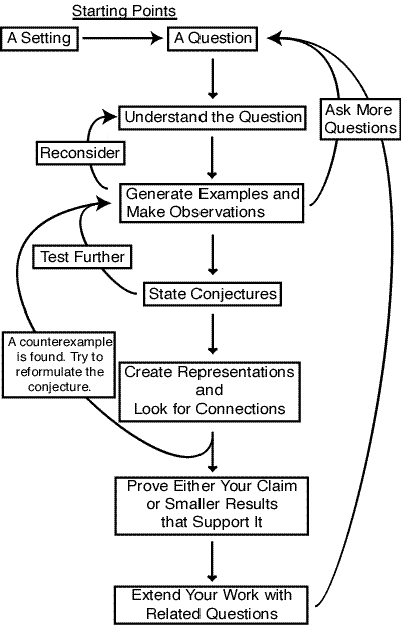Figure 1. The Research Process.

## HILGEMEIER’S LIKENESS SEQUENCE

This activity introduces many of the overarching ideas of mathematics research in a two- or three- period sequence. It uses a setting rather than a question so that students will be encouraged to pose a range of mathematical questions.

Software is available for further explorations and testing of conjectures.

### Goals for This Activity

• To introduce some of the stages of doing mathematics research: understanding a problem, having a question, collecting and organizing data, making a conjecture, and generalizing a question
• To broaden students’ understanding of what counts as a mathematical question or setting
• To teach students to distinguish between an example, a conjecture, and a theorem
• Optional: To start considering the nature of proof and the group process of evaluating the reasoning behind a claim

### Suggested Pacing:

 Lesson In-Class Homework 1 Understanding the problem and posing questions Extending the sequence and the list of questions 2 Collecting data in response to the questions Stating conjectures Generating some early answers and explanations Testing conjectures and informal proofs 3 Discussing the conjectures and the role of evidence and reasoning Optional: Further explorations with the program SequenceGenerator

### The Sequence

1

11

21

12, 11

11, 12, 21*

31, 22, 11

13, 11, 22, 21

11, 13, 21, 32, 11

* Read in pairs for describing the line above, but read as 111, 22, 1 when described by the line below.

Figure 2. The First Eight Terms of Hilgemeier’s Likeness Sequence

This lesson revolves around Hilgemeier’s Likeness Sequence (see Figure 2, above), which is generated according to the following rule: The first line is an arbitrary starting point, and each line thereafter is a description of the string of digits in the prior line, read left to right. For example, the third line reads "Two 1’s," which is a description of the second line. The fifth line reads "One 1, one 2, two 1’s," which describes the line before it, grouping consecutive strings of identical digits.

### Introduction

Explain to the class that you are going to present a sequence, each term of which is a sequence of numbers. Ask them to study it and try to determine how it is generated, what properties it may have, and what patterns it may exhibit. Display the first five lines (see Figure 3 below) and ask students to copy them and then spend three minutes trying to anticipate the next term in the sequence.

1

11

21

1211

111221

Figure 3. The First Five Terms of Hilgemeier’s Likeness Sequence

As students study the terms, circulate and listen for questions or observations (e.g., "The lines are getting longer," or "Are there only 1’s and 2’s?"). Write these on the board. Solicit students’ guesses and explanations for the next term. These guesses will reveal for the class the kinds of patterns that might be noticed, for example, that there always seems to be a "1" at the end of a term.

Write the next few terms on the board (see Figure 4, below), pausing after each to note any stray comments worth adding to the board (e.g., "Aha, a 3! Will a 4 come next?").

312211

13112221

1113213211

31131211131221

13211311123113112211

Figure 4. Terms 6 through 10

It is quite difficult to unravel the rule behind this sequence. The purpose of this gradual revelation is to bring out how the class thinks about numerical patterns and to list their initial observations and questions about the sequence’s behavior. It is fine if they do not figure out the rule themselves (and the mild frustration they experience is also a gentle introduction to the pace of real mathematics investigations).

After 10 or so minutes of (most likely) unsuccessful searching for a rule that explains the full sequence, add a few more lines, add commas between the pairs (see Figure 5, below), and read the first few lines out loud, given this punctuation (the fifth line should be read "One one <pause> one two <pause> two one" not "eleven, twelve, twenty-one").

1

11

21

12, 11

11, 12, 21

31, 22, 11

13, 11, 22, 21

Figure 5. The First Seven Terms of the Sequence, Punctuated

Once students have generated a few questions and observations about the sequence, you can add plurals to your reading of the list: "Three ones <pause> two twos <pause> one one." If a student thinks he or she has figured out the pattern, ask the student for the next line without telling the class the rule. (This is mostly for dramatic effect, but the drama serves to add to the surprise and outrage, both of which have value.) If the student generates a correct line, have her or him reveal the rule; otherwise, give someone else a chance. If no one figures it out, tell the class how each line is generated.

### Where’s the Math?

Often a student will object, either vociferously or muttered, "This is supposed to be math class, not English!" This protestation is your cue to point to the list of questions and observations on the board. Is "Will a 4 appear?" an English question or a mathematical one? Acknowledge that the sequence is a rather odd mathematical object, but it is one, and it led the class to raise interesting mathematical observations and questions. The absence of an arithmetic rule for the sequence did not exhaust the range of possible mathematical explanations. Point out that mathematics is about patterns, structures, and reasoning, and that we are not limited to numbers and shapes as the subject of our studies. (In this case, the digits are playing dual roles as numbers, if they are the first part of a pair, and symbols, if they are the second part.)

In addition to noting the terminal "1" of each term and wondering about the appearance of a "4", other questions students have asked during the early stage of this activity include the following:

• Is the length of each term in the sequence always going to be the same or greater than its predecessor (monotonically increasing)? The class introduced the notation L(n) for the number of digits in the nth term and rephrased this question symbolically: Is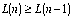for all counting numbers n?
• Is there an explicit formula for the sequence? (This student sensed the recursive nature of the problem and wondered whether one needed a given line to generate the subsequent term.)
• Will the second-to-last digit always alternate?

Have everyone write down the next three or more lines of the sequence. Make sure they understand the rule. Give the students seven minutes (see Assessment and the Use of Class Time for an explanation of this specific interval of time) to write additional lines, test any of the current questions, or pose some new questions about the sequence. Encourage them to check their new terms with a partner.

### Mathematical Statements

When the class reconvenes, solicit any new questions and add them to the list. Provide the following definitions informally: The individual terms each serve as an example of what terms in the sequence might be like. They also have a relationship to one another in establishing broader patterns. The class saw the sequence and noted, "Hmm, the digits do not seem to be going beyond 3." Such a comment is an observation, based on several examples, that reflects a general pattern or property of the objects under investigation. If, after further exploration and consideration, we begin to believe an observation will always hold true, then we state it clearly and call it a conjecture. The question "Does a 4 appear?" might be turned into the conjecture "A 4 will not appear in this sequence." A conjecture is a claim that we believe is likely to be true but have not yet proven true. Once a conjecture is proven, it is called a theorem.

Ask students if they have thoughts about how to answer any of the questions posed. If none are proffered, prompt them with questions such as, "What would they do to find out if a 4 appears?" or "How could you determine if the final two digits continue their 11, 21, 11, 21, … pattern?" The goal of these questions is not to push students into rigorous proofs, but to distinguish between the role that different types of evidence play in mathematical thinking. If a student suggests that the class extend the sequence in order to verify a conjecture, have the class put additional terms on the board and ask whether these new terms answer any of their questions. Should a continuation of the pattern convince them that a 4 will never appear? (Many may say yes.) What would they require to be sure that the conjecture was true or false? Students may suggest that its truth merely requires a single example, a term with a 4 in it. (The truth of the conjecture might also be shown through an existence proof, which does not give a particular term but is able to show that, for some reason, a 4 must ultimately appear. This approach can be left for a later discussion.) Could examples ever prove the conjecture false? Many more terms might appear persuasive and make us ever more supportive of our conjecture, but a claim about an infinite set of elements cannot be proven with a finite sample (note that the claim that a 4 does appear may be a claim about only a single term; see the activity Conjectures Are Not Theorems in the Examples, Patterns, and Conjectures section).

### Homework

The first homework assignment is dependent on how far the discussion progressed during class. Students might be asked to extend the sequence. They should be asked to generate at least three new questions about the sequence. They can also be given the option of trying to answer any questions that have not yet been addressed.

The sophistication of the questions students ask about the sequence tends to grow as they immerse themselves in the setting. Here are some questions that students have posed during later stages of this investigation:

• The lengths of the fifth and sixth terms are equal: L(5) = L(6). Will L(n) = L(n – 1) an infinite number of times? Will L(n) = L(n – 1) ever again for n > 6? If so, will there be a pattern to the values of n for which adjacent terms are equal?
• Is there a formula for L(n)? Is its growth a familiar function (linear, exponential)?
• Does the sequence have any application?
• What happens if you start with a different seed (e.g., with a 2 or a 7 instead of a 1 in the first line)?

The last question in this list is wonderful. It is not a question about the specific sequence, but about the process involved. It is the start of a generalization of the original setting. All of the other questions can be applied to this new sequence (2, 12, 1112, 3112, 132112, etc.) and their answers in each case compared. When students take the initiative to generalize or modify a research problem, their contribution should be highlighted as an example of the broader context in which research questions can and should be viewed (see Problem Posing).

Once the question list has grown, it is worth reminding the class that any initial skepticism about the mathematical nature of the problem should be gone. In fact, by this point, students are more likely to be surprised at the amount of time it would take to even try to resolve the questions.

At this juncture, students can individually or in pairs choose one of the questions to investigate further. Students can study the growth of L(n) or the appearance of patterns in the terms themselves with the program SequenceGenerator. Students can turn some of their questions into conjectures and try to come up with reasons (other than a preponderance of examples) for believing them (see Sample Student Proofs, below).

The emphasis at this point should not be on rigorous proof but on clear communication, group understanding of the conjectures and reasons that students produce, and understanding the relationship between data, conjectures, and theorems.

Whether further time is devoted to exploring this sequence or extensions of the setting should be based on class enthusiasm and the likely productivity of the questions raised. Finding a function for L(n) by transforming familiar functions (polynomial and exponential) or with curve-fitting algorithms (such as are built into most graphing calculators) might be of interest, but an attempt to find a proof of this behavior is not a constructive use of time. If the class is enjoying the questions, spending further time exploring them will send the message that their mathematical enthusiasm is appropriate and encouraged (see Student and Teacher Affect).

## SAMPLE STUDENT PROOFS (Paraphrased)

These proofs are approaches that inspired students developed to support their conjectures about the sequence. They are provided for your interest, rather than as examples of what a typical class produces. Note that while even arithmetic is barely necessary, the reasoning did require careful keeping track of possible cases and encouraged the introduction of symbols. See Reading Technical Literature for suggestions on wading through dense mathematical reasoning. Proofs of some of the claims about this sequence require both elaborate case-by-case schemes (see Conway's Constant and its links to Conway’s "periodic table") and more complex mathematics.

This introductory activity need not lead to proofs and should not be used as the point to insist on perfect form and rigor. If a student does present a proof, encourage the class to study it, check its claims, and offer constructive feedback. Are the explanations clear? Convincing? Do they cover all possibilities? Use this opportunity to discuss the value of proof (see Proof and Proof Techniques) and note whether the arguments your students present offer insight into why their theorem is true (as the two below do).

### First Student Proof

Can the original sequence produce a 4? No.

The digits in a term either refer to a symbol (S) in the previous term or are a count (C) of how many times such a symbol appeared consecutively. Since there is no 4 to begin with, the first appearance of a 4 must come from there being four consecutive appearances of some other symbol. The terms are made of pairs of digits: C1S1C2S2 . . . CnSn. Any string of four of these must include two adjacent CS pairs all of the same digit (either . . . CiSiCi+1Si+1 . . . or . . . SiCi+1Si+1Ci+2 . . .). A term cannot have the same value for S twice in a row because you are counting strings of symbols until a new string begins (if 2111 was describing three symbols in a preceeding line, it should just be 31). So four identical symbols would never appear consecutively and therefore a 4 will not appear.

### Second Student Proof

Can L(n) be less than L(n – 1)? Not for n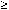3.

There are three types of CS pairs in the terms of the sequence. The Ci can equal either 1, 2, or 3.

CS pairs beginning with a 1 are one digit longer than the substring they describe.

CS pairs beginning with a 2 are the same length as the substring they describe.

CS pairs beginning with a 3 are one digit shorter than the substring they describe. Replacing a string of three identical digits with a pair, such as 32, is the only way a term can shrink.

So L(n) can be less than L(n – 1) only if there are more CS pairs beginning with a 3 than there are pairs beginning with a 1.

Any string of three consecutive digits must begin at a C value (or you will have two consecutive, identical S values). We are trying to see if we can fit in more three-digit substrings than one-digit ones, so let’s begin a term with a three-digit string: aaa. The fourth digit of this term must be different (aaab) and cannot be part of a three-digit string (for the two S reason), so we have aaabc . . . or aaabb . . . In the aaab case, the one b will produce a two digit descriptive CS pair and so aaab will be replaced by the equally long 3a1b substring. In the latter cases, we have one fewer digit, but we began with a five-digit term and odd length terms are only possible as our starting term (So L(2) can be shorter than L(1) but not thereafter). If n > 2, then the nth term is describing an even-length term and the aaabb… substring must be followed either by a terminating single digit (which yields a descriptive pair and cancels out the "benefit" of the initial triple) or by another pair, until some final single digit. Not until a single digit has been added to get a starting substring that can begin in a C position, can a new triple be appended. So, each triple must be followed by a complementary single (with or without an intervening sequence of pairs), and it is impossible to have more triples than singles. Therefore, L(n) must be at least as long as L(n – 1).

### Extensions

Another question students may consider is, "Can three 3’s appear in a sequence?"

An extension students may explore is Robinsonizing, which poses this question: What if each line tells, in order, how many total 1’s, 2’s, 3’s, etc. appear in the prior line (with no attention paid to the order of the digits). For example:

0 1’s, 0 2’s, 0 3’s, 0 4’s, 0 5’s, 0 6’s, 0 7’s, 0 8’s, 0 9’s, 0 0’s

1 1’s, 1 2’s, 1 3’s, 1 4’s, 1 5’s, 1 6’s, 1 7’s, 1 8’s, 1 9’s, 11 0’s

12 1’s, 1 2’s, 1 3’s, 1 4’s, 1 5’s, 1 6’s, 1 7’s, 1 8’s, 1 9’s, 1 0’s

11 1’s, 2 2’s, 1 3’s, 1 4’s, 1 5’s, 1 6’s, 1 7’s, 1 8’s, 1 9’s, 1 0’s

How does this sequence continue? What happens if we choose a different starting string? (See Douglas Hofstadter’s Metamagical Themas , pages 27 and 390–92 for more information.)

## BIBLIOGRAPHY

Berzsenyi, George (1993, Sept./Oct.). Endless self description: Finding the limits in Hilgemeier’s "likeness sequence." Quantum, 17.

Conway's Constant (2000). Available online at http://www.mathsoft.com/asolve/constant/cnwy/cnwy.html.

Conway, John (1990, Nov./Dec.). Play it again… Quantum, 31, 63.

Hofstadter, Douglas (1985). Metamagical themas. New York: Basic Books.

Look and say sequence in Weisstein, Eric (1999). Concise encyclopedia of mathematics CD-ROM. USA: CRC Press. Also available online at http://mathworld.wolfram.com/LookandSaySequence.html.

## APPENDIX

Encourage students with a programming background to create an application that generates the terms of the Likeness Sequence. Alternatively, you can provide your class with the following Logo programs to use in their explorations. A program makes it possible to study terms of the sequence that are much longer than students can reasonably study by hand. See http://el.www.media.mit.edu/groups/logo-foundation/products/software.html for more information about Logo programs, including some that can be downloaded for free.

The SeeAndSay procedure accepts a list as input and produces the list that describes it. For example SeeAndSay [3 8 8 1] outputs [1 3 2 8 1 1]. The SequenceGenerator procedure takes a starting list and the number of lines to be displayed and generates the first n lines beginning with that initial list. For example, SequenceGenerator [3 2] 5 produces:

3 2

1 3 1 2

1 1 1 3 1 1 1 2

3 1 1 3 3 1 1 2

1 3 2 1 2 3 2 1 1 2

to SeeAndSay :list

if :list = [] [output []]

if (butfirst :list) = [] [output se "1ist first :list]

if not ((first :list) = first butfirst :list) [output (se "1ist first :list SeeAndSay butfirst :list)]

if and ((first :list) = first butfirst :list) ((butfirst butfirst :list) = []) [output (se "2 first :list SeeAndSay butfirst butfirst :list)]

if and ((first :list) = first butfirst :list) ((first :list) = first butfirst butfirst :list) [output (se "3 first :list SeeAndSay butfirst butfirst butfirst :list)]

output (se "2 first :list SeeAndSay butfirst butfirst :list)

end

to SequenceGenerator :list :n

print :list

if :n > 1 [SequenceGenerator SeeAndSay :list :n - 1]

end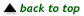Translations of mathematical formulas for web display were created by tex4ht. © Copyright 2003 Education Development Center, Inc. (EDC)